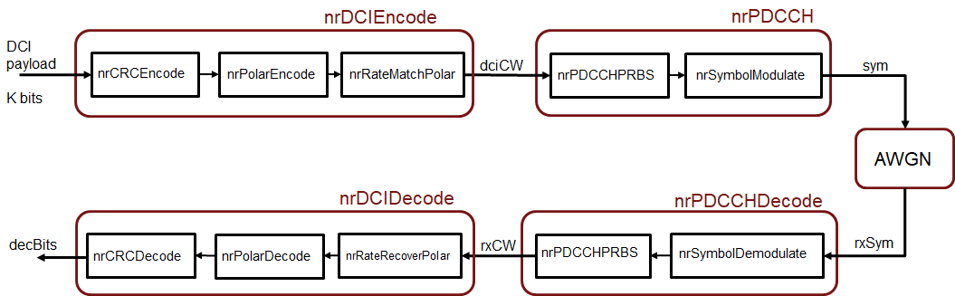This example describes the downlink control information (DCI) processing for the 5G New Radio communications system. Starting from a random DCI message, it models the message encoding followed by the physical downlink control channel (PDCCH) processing on the transmit end. Corresponding receiver components recover the transmitted control information elements.

System Parameters

Set parameters for a UE-specific search space.

rng(211);           % Set RNG state for repeatability

nID = 23;           % pdcch-DMRS-ScramblingID
rnti = 100;         % C-RNTI for PDCCH in a UE-specific search space
K = 64;             % Number of DCI message bits
E = 288;            % Number of bits for PDCCH resources

DCI Encoding

The DCI message bits based on a downlink format are encoded using the nrDCIEncode function, which includes the stages of CRC attachment, polar encoding and rate matching.

dciBits = randi([0 1],K,1,'int8');
dciCW = nrDCIEncode(dciBits,rnti,E);

PDCCH Symbol Generation

The encoded DCI bits (a codeword) are mapped onto the physical downlink control channel (PDCCH) using the nrPDCCH function which generates the scrambled, QPSK-modulated symbols. The scrambling accounts for the user-specific parameters.

sym = nrPDCCH(dciCW,nID,rnti);

For NR, the PDCCH symbols are then mapped to the resource elements of an OFDM grid which also has PDSCH, PBCH and other reference signal elements. These are followed by OFDM modulation and transmission over a channel. For simplicity, we directly pass the PDCCH symbols over an AWGN channel next.

The following schematic depicts the components used in the example for DCI processing.Channel

The PDCCH symbols are transmitted over an AWGN channel with a specified SNR, accounting for the coding rate and QPSK modulation.

EbNo = 3;                       % in dB
bps = 2;                        % bits per symbol, 2 for QPSK
EsNo = EbNo + 10*log10(bps);
snrdB = EsNo + 10*log10(K/E);

rxSym = awgn(sym,snrdB,'measured');

PDCCH Decoding

The received symbols are demodulated with known user-specific parameters and channel noise variance using the nrPDCCHDecode function. The soft output is the log-likelihood ratio for each bit in the codeword.

noiseVar = 10.^(-snrdB/10);     % assumes unit signal power
rxCW = nrPDCCHDecode(rxSym,nID,rnti,noiseVar);

DCI Decoding

An instance of the received PDCCH codeword is then decoded by the nrDCIDecode function. This includes the stages of rate recovery, polar decoding and CRC decoding to recover the transmitted information bits.

listLen = 8;                    % polar decoding list length

ans = logical
1

isequal(decDCIBits,dciBits)
ans = logical
1

For a known recipient, the C-RNTI information aids decoding. The output mask value of 0 indicates no errors in the transmission. For the chosen system parameters, the decoded information matches the transmitted information bits.